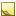﻿Weight data typeWeight data type

The data type Weight represents a weight measurement.

The TDE data types for quantifiable data (numeric values with units of measure) are all stored as double and are handled similarly fortemplate scripting.Tip

Since every quantifiable data type is stored as double, be sure to account for that when you test equivalency (for example, 1 may actually be stored as 0.999999). Either round off the values being compared, or use ranges for the comparison.Units
• kilogram or kg — default

• gram or g

• pound or lb

Data from TDE defaults to the first unit listed for each data type. In the [Output] section, you can convert these values to the units you need:

```[Output]
MaxPounds(lb) = input.MaxWeight   # converts km to pounds```Field length

The effective range of this data type is: 0 to 1,000,000,000 kgJavaScript conversion

When you use the optional [Script] section to insert your own JavaScript functions, access this data type as follows:

Constructor
JavaScript
`new Weight({Unit:Value})`
Available fields for (get,set)

You can access the valid units of measure as fields:

• .kilogram

• .gram

• .pound